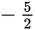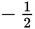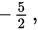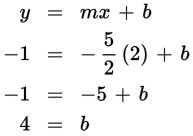# SAT Math Multiple Choice Question 437: Answer and Explanation

### Test Information

Question: 437

2. If the slope of a line isand a point on the line is (2, –1), which of the following is the y-intercept of the line?

• A. -6
• B.• C. 4
• D. 6

Explanation:

C

Difficulty: Medium

Category: Heart of Algebra / Linear Equations

Strategic Advice: When you know the slope and one point on a line, you can use y = mx + b to write the equation. Substitute the slope for m and the coordinates of the point for x and y. Then solve for b, which gives you the y-intercept of the line.

Getting to the Answer: The slope is given asso substitute this for m. The point is given as (2, –1), so x = 2 and y = –1. Now, find b.The y-intercept of the line is 4.

You could also very carefully graph the line using the given point and the slope. Start at (2, –1) and move toward the y-axis by rising 5 and running to the left 2 (because the slope is negative). You should land at the point (0, 4).